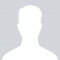Class Central is learner-supported. When you buy through links on our site, we may earn an affiliate commission.

# Practical Numerical Methods with Python

### Overview

This course is a collaboration between faculty at three institutions across the world: the George Washington University (Washington, DC, USA); University of Southampton (UK) and Pontifical Catholic University of Chile (Santiago, Chile).* A credit-bearing course will run at each institution at the same time as this MOOC, and students at all locations will participate in the same learning community with MOOC participants.

The course aims for students to achieve the following:

• connect the physics represented by a mathematical model to the characteristics of numerical methods to be able to select a good solution method;
• implement a numerical solution method in a well-designed, correct computer program;
• interpret the numerical solutions that were obtained in regards to their accuracy and suitability for applications.

WHO IS THE COURSE FOR?

Numerical methods for differential equations are relevant across all of science and engineering. This course is for anyone with mathematical, scientific or engineering backgrounds who wishes to develop a grounding in scientific computing. Using a range of hands-on lessons, participants in the course will develop the basic skills to tackle modern computational modelling problems.

In developing this course, the instructors are inspired by the philosophy of open-source software. One of the tenets of the course is that we can use the web to interact, connect our learning, teach each other by sharing our learning objects. Therefore, this course is especially for those who are eager to participate in distributed knowledge creation on the web. Join us in this adventure!

PREREQUISITES

The connected courses and MOOC are aimed at first-year graduate students or advanced seniors, but assume only a background in vector calculus, linear algebra, and differential equations. We won't assume more than a beginner's programming experience and will guide students to develop a foundation in numerical methods, and hands-on experience coding up solutions to differential equations.

### Syllabus

The course will consist of six learning modules that are somewhat self-contained. Each one will be motivated by a problem that can be modeled by a differential equation (or system of DEs) and will build new concepts in numerical computing, new coding skills and ideas about analysis of numerical solutions.

The learning modules are roughly as follows:

The phugoid model of glider flight.

Described by a set of two nonlinear ordinary differential equations, the phugoid model motivates numerical time integration methods, and we will build it starting from an even simpler model (e.g., simple harmonic motion), building up to the full nonlinear model in 4 or 5 lessons on initial-value problems. Roughly, this module would include: a) Forward/backward differencing and Euler's method for simple harmonic motion; b) extension to the phugoid model; c) the midpoint method, convergence testing, local vs. global error; d) Runge-Kutta methods.

Space and Time—Introduction to finite-difference solutions of PDEs

Starting with the simplest model represented by a partial differential equation (PDE)—the linear convection equation in one dimension—, this module builds the foundation of using finite differencing in PDEs. (The module is based on the “CFD Python” collection, steps 1 through 4.) It also motivates CFL condition, numerical diffusion, accuracy of finite-difference approximations via Taylor series, consistency and stability, and the physical idea of conservation laws.

Riding the wave: convection problems.

Starting with the inviscid Burgers’ equation in conservation form and a 1D shock wave, cover a sampling of finite-difference convection schemes of various types: upwind, Lax-Friedrichs, Lax-Wendroff, MacCormack, then MUSCL (discussing limiters). Traffic-flow equation with MUSCL (from HyperPython). Reinforce concepts of numerical diffusion and stability, in the context of solutions with shocks. It will motivate spectral analysis of schemes, dispersion errors, Gibbs phenomenon, conservative schemes.

Start with heat equation in 2D (first introduction of two-dimensional FD discretization). Introduce implicit methods: backward Euler, trapezoidal rule (Crank-Nicolson), backward-differentiation formula (BDF). Pattern formation models (reaction-diffusion). Theory content: A-stability (unconditional stability), L-stability. Fourier spectral methods and splitting.

Relax and hold steady: elliptic problems.

Laplace and Poisson equations (steps 9 and 10 of “CFD Python”), explained as systems relaxing under the influence of the boundary conditions and the Laplace operator; introducing the idea of pseudo-time and iterative methods. Linear solvers for PDEs : Jacobi’s method, slow convergence of low-frequency modes (matrix analysis of Jacobi), Jacobi as a smoother, Multigrid.

Boundaries take over: the boundary element method (BEM)

Weak and boundary integral formulation of elliptic partial differential equations; the free space Green's function. Boundary discretization: basis functions; collocation and Galerkin systems. The BEM stiffness matrix: dense versus sparse; matrix conditioning. Solving the BEM system: singular and near-singular integrals; Gauss quadrature integration.

### Taught by

David Ketcheson, Carlos Jerez, Ian Hawke and Lorena A. Barba

## Reviews

4.0 rating, based on 4 Class Central reviews

Start your review of Practical Numerical Methods with Python

• Jonah Miller completed this course, spending 3 hours a week on it and found the course difficulty to be medium.

Sans videos, an innovative and interactive approach to an introductory Python course that assumes very little previous programming experience but must have some background in vector calculus, linear algebra, and differential equations.
• Anonymous

Anonymous completed this course.

I came into this course knowing absolutely no Python and by the end of it, I was proficient in the language and able to utilize it for numerical analysis. In fact, I have come to see Python as a more powerful and more intuitive tool than even MATLA…
•Alex Ivanov

Alex Ivanov completed this course.

• Anonymous

Anonymous is taking this course right now.

### Never Stop Learning.

Get personalized course recommendations, track subjects and courses with reminders, and more.